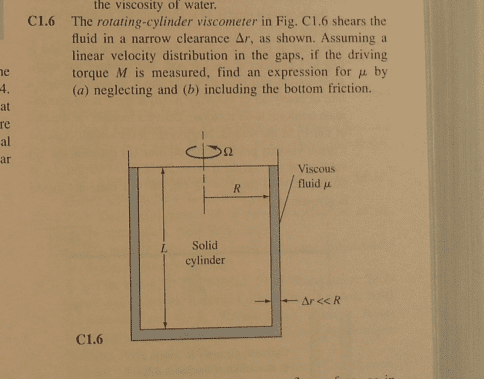# Fluid Mechanics: Rotating cylinder viscometer

• Feodalherren

## Homework Statementτ = μ (du/dy)

## The Attempt at a Solution

For part B)

dM = τr dA

τ = μ (rΩ/Δr)

∫ (μrΩr2πr / Δr)dr = μR4πΩ / 2Δr

pretty sure that's correct.

I'm confused for part A though.

I want to set it up as

dM = τR dA = τR (2πRdL)

and

τ = μ (RΩ/L)

substitute and integrate over 0 to L. But this doesn't yield the correct result.

Part A is done incorrectly. The velocity gradient in the gap is ##\frac{ΩR}{Δr}##. So the shear stress is ##μ\frac{ΩR}{Δr}##

Chet

•Feodalherren
Ahh of course. It's so obvious now. Thank you, Sir.

Would that mean that part A becomes:

dM = τR dA = τR (2πRdL)

M = ∫ R(2πRdL) (ΩR/Δr) = 2πR3ΩLμ / Δr

correct?

Ahh of course. It's so obvious now. Thank you, Sir.

Would that mean that part A becomes:

dM = τR dA = τR (2πRdL)

M = ∫ R(2πRdL) (ΩR/Δr) = 2πR3ΩLμ / Δr

correct?
Yes.

•Feodalherren
Thanks!